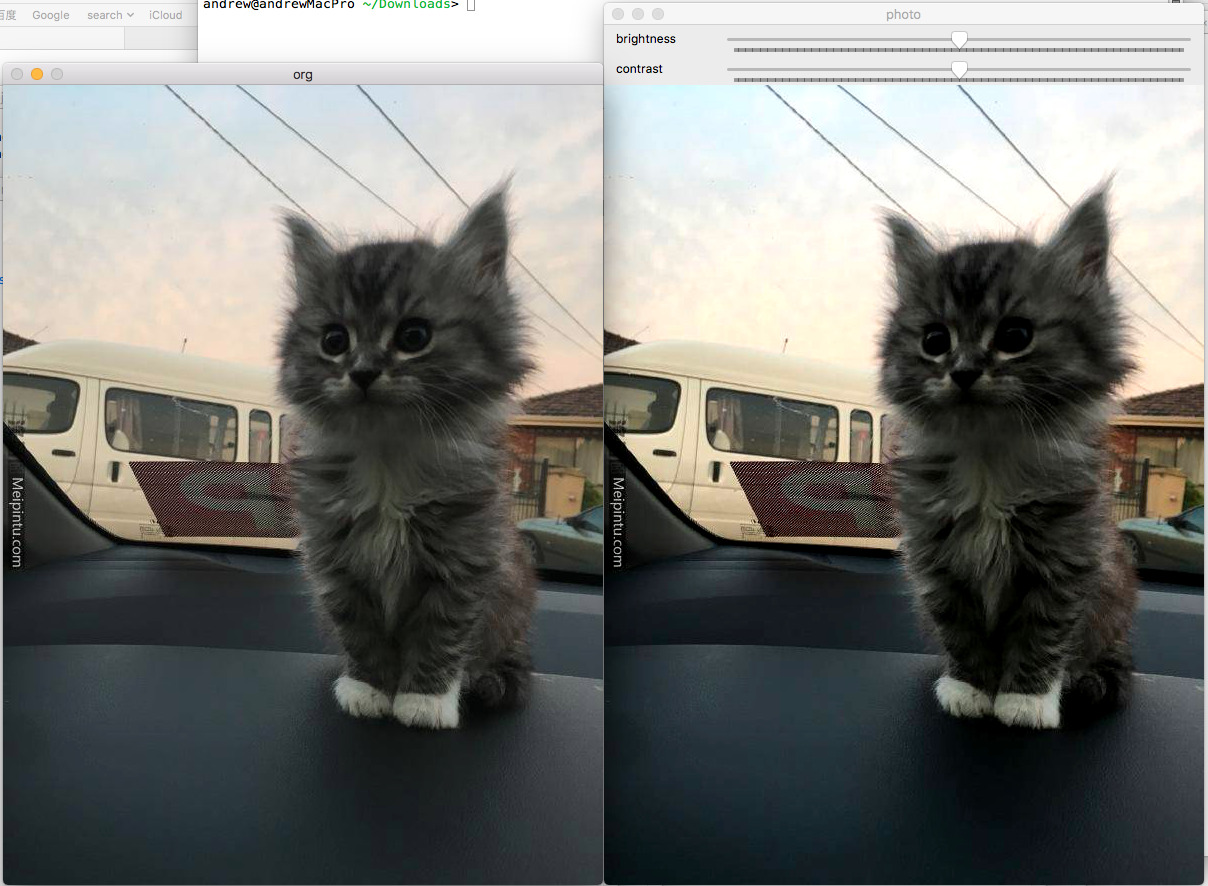# OpenCV中的亮度对比度调整及其自动均衡

f = a*X+b

#### 什么样算好？（国产电视剧《琅琊榜》剧照）（《星球大战》某前传剧照）

#### 亮度对比度的自动均衡``````	1.把彩色图转换成灰度图，因为亮度对比度调整不涉及偏色的调整。
2.统计灰度图每一个色值的数量。
3.min=最小的非0值。
4.max=最大的非255值。
5.使用灰度图计算出来的min/max值，把彩色图min-max之间的数据重新分布于0-255的范围。
``````

#### 源码

``````#include <iostream>
#include "opencv2/core.hpp"
#include "opencv2/imgproc.hpp"
#include "opencv2/highgui.hpp"

using namespace std;
using namespace cv;

#define CLIP_RANGE(value, min, max)  ( (value) > (max) ? (max) : (((value) < (min)) ? (min) : (value)) )
#define COLOR_RANGE(value)  CLIP_RANGE(value, 0, 255)

/**
*  \brief Automatic brightness and contrast optimization with optional histogram clipping
*  \param [in]src Input image GRAY or BGR or BGRA
*  \param [out]dst Destination image
*  \param clipHistPercent cut wings of histogram at given percent tipical=>1, 0=>Disabled
*  \note In case of BGRA image, we won't touch the transparency
*/
void BrightnessAndContrastAuto(const Mat &src, Mat &dst, float clipHistPercent=0)
{
CV_Assert(clipHistPercent >= 0);
CV_Assert((src.type() == CV_8UC1) || (src.type() == CV_8UC3) || (src.type() == CV_8UC4));

int histSize = 256;
float alpha, beta;
double minGray = 0, maxGray = 0;

//to calculate grayscale histogram
Mat gray;
if (src.type() == CV_8UC1) gray = src;
else if (src.type() == CV_8UC3) cvtColor(src, gray, CV_BGR2GRAY);
else if (src.type() == CV_8UC4) cvtColor(src, gray, CV_BGRA2GRAY);
if (clipHistPercent == 0)
{
// keep full available range
minMaxLoc(gray, &minGray, &maxGray);
}
else
{
Mat hist; //the grayscale histogram

float range[] = { 0, 256 };
const float* histRange = { range };
bool uniform = true;
bool accumulate = false;
calcHist(&gray, 1, 0, Mat(), hist, 1, &histSize, &histRange, uniform, accumulate);

// calculate cumulative distribution from the histogram
vector<float> accumulator(histSize);
accumulator = hist.at<float>(0);
for (int i = 1; i < histSize; i++)
{
accumulator[i] = accumulator[i - 1] + hist.at<float>(i);
}

// locate points that cuts at required value
float max = accumulator.back();
clipHistPercent *= (max / 100.0); //make percent as absolute
clipHistPercent /= 2.0; // left and right wings
// locate left cut
minGray = 0;
while (accumulator[minGray] < clipHistPercent)
minGray++;

// locate right cut
maxGray = histSize - 1;
while (accumulator[maxGray] >= (max - clipHistPercent))
maxGray--;
}

// current range
float inputRange = maxGray - minGray;

alpha = (histSize - 1) / inputRange;   // alpha expands current range to histsize range
beta = -minGray * alpha;             // beta shifts current range so that minGray will go to 0

// Apply brightness and contrast normalization
// convertTo operates with saurate_cast
src.convertTo(dst, -1, alpha, beta);

// restore alpha channel from source
if (dst.type() == CV_8UC4)
{
int from_to[] = { 3, 3};
mixChannels(&src, 4, &dst,1, from_to, 1);
}
return;
}

/**
* Adjust Brightness and Contrast
*
* @param src [in] InputArray
* @param dst [out] OutputArray
* @param brightness [in] integer, value range [-255, 255]
* @param contrast [in] integer, value range [-255, 255]
*
* @return 0 if success, else return error code
*/
int adjustBrightnessContrast(InputArray src, OutputArray dst, int brightness, int contrast)
{
Mat input = src.getMat();
if( input.empty() ) {
return -1;
}

dst.create(src.size(), src.type());
Mat output = dst.getMat();

brightness = CLIP_RANGE(brightness, -255, 255);
contrast = CLIP_RANGE(contrast, -255, 255);

/**
Algorithm of Brightness Contrast transformation
The formula is:
y = [x - 127.5 * (1 - B)] * k + 127.5 * (1 + B);

x is the input pixel value
y is the output pixel value
B is brightness, value range is [-1,1]
k is used to adjust contrast
k = tan( (45 + 44 * c) / 180 * PI );
c is contrast, value range is [-1,1]
*/

double B = brightness / 255.;
double c = contrast / 255. ;
double k = tan( (45 + 44 * c) / 180 * M_PI );

Mat lookupTable(1, 256, CV_8U);
uchar *p = lookupTable.data;
for (int i = 0; i < 256; i++)
p[i] = COLOR_RANGE( (i - 127.5 * (1 - B)) * k + 127.5 * (1 + B) );

LUT(input, lookupTable, output);

return 0;
}

void waitESC(){
while(int key=waitKey(0) != 27){};
}

static string window_name = "photo";
static Mat src,src1,dst1;
static int brightness = 255;
static int contrast = 255;

static void callbackAdjust(int , void *)
{
Mat dst;
adjustBrightnessContrast(src, dst, brightness - 255, contrast - 255);
imshow(window_name, dst);
}

int main(int argc,char **argv){

if (argc != 2) {
printf("%s imgfile",argv);
}
imshow("org",src1);
BrightnessAndContrastAuto(src1,src,5);

if ( !src.data ) {
cout << "error read image" << endl;
return -1;
}

namedWindow(window_name);
createTrackbar("brightness", window_name, &brightness, 2*brightness, callbackAdjust);
createTrackbar("contrast", window_name, &contrast, 2*contrast, callbackAdjust);

waitESC();

return 0;

}

``````参考链接：
http://blog.csdn.net/c80486/article/details/52505061